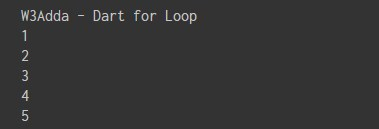# Dart for Loop

In this tutorial you will learn about the Dart for Loop and its application with practical example.

## Dart for Loop

The for loop is used when we want to execute block of code known times. In Dart, basic for loop is similar as it is in C, C++ and Java. The for loop takes a variable as iterator and assign it with an initial value, and iterate through the loop body as long as the test condition is true. Once the loop statements are executed for current iteration, the iterator is updated with new value and if the test condition is still valid, we loop another time. When test condition return a false value, the for loop is ended.

Syntax :-

Above is the general syntax of a for loop. We just initialize iterator, check condition and then increment or decrement iterator value. The for loop has three components separated by semicolons.

initialization :- In this section is used to declare and initialize the loop iterator. This is the first statement to be executed and executed only once at the beginning.

condition :- Next, the test condition is evaluated before every iteration, and if it returns a true value then the loop body is executed for current iteration. If it returns a false value then loop is terminated and control jumps to the next statement just after the for loop.

incr/decr :- Once, the loop body is executed for current iteration then the incr/decr statement is executed to update the iterator value and if the test condition is evaluated again. If it returns a true value then the loop body is executed another time. If test condition returns a false value then loop is terminated and control jumps to the next statement just after the for loop.

## Dart For Loop Flow DiagramExample:-

Here, we have initialized ctr with initial value of 1, in the next we checks the condition ctr <= 5 for each of the iteration. If the test condition returns True, the statements inside loop body are executed and if test condition returns False then the for loop is terminated. In incr/decr section we have incremented the ctr value by one for each if the iteration. When we run the above Dart program, we see the following output –

Output:-In this tutorial we have learn about the Dart for Loop and its application with practical example. I hope you will like this tutorial.